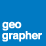# Count topology errors in Python

2453
2
10-22-2014 07:02 AMOccasional Contributor

Hi,

Are there any hooks to get the count of errors in a topology via Arcpy? Using Describe only returns information about the Topology settings, not the number of errors, types, etc.

TIA,

Greg

Tags (3)
1 Solution

Accepted SolutionsOccasional Contributor

I figured this out: I converted all of the topologies to feature classes using ExportTopologyErrors_management() and then counted the resulting feature class features. Code is below. The topologies were generated by a script across File GDBS, so I'm relying on the naming convention to process them all.

```import arcpy, os
from arcpy import env
outws = r'D:\temp\topocount.gdb'
env.workspace = r'D:\data'
fgdbs = arcpy.ListWorkspaces('*','FileGDB')
for fgdb in fgdbs:
env.workspace = fgdb
dataset = arcpy.ListDatasets('*','Feature')
topology = os.path.join(dataset, dataset + '_topology')
topology = os.path.join(fgdb, topology)
print 'Converting %s' %topology
arcpy.ExportTopologyErrors_management(topology,outws,dataset + '_')

print 'Counting topology errors.'
# count 'em up
with open(r'D:\temp\topology_error_count.txt', 'w+') as f:
env.workspace = outws
fcs = arcpy.ListFeatureClasses()
for fc in fcs:
count = arcpy.GetCount_management(fc)
print count
outStr = fc + ',' + str(count) + '\n'
f.writelines(outStr)

```
2 RepliesOccasional Contributor

I figured this out: I converted all of the topologies to feature classes using ExportTopologyErrors_management() and then counted the resulting feature class features. Code is below. The topologies were generated by a script across File GDBS, so I'm relying on the naming convention to process them all.

```import arcpy, os
from arcpy import env
outws = r'D:\temp\topocount.gdb'
env.workspace = r'D:\data'
fgdbs = arcpy.ListWorkspaces('*','FileGDB')
for fgdb in fgdbs:
env.workspace = fgdb
dataset = arcpy.ListDatasets('*','Feature')
topology = os.path.join(dataset, dataset + '_topology')
topology = os.path.join(fgdb, topology)
print 'Converting %s' %topology
arcpy.ExportTopologyErrors_management(topology,outws,dataset + '_')

print 'Counting topology errors.'
# count 'em up
with open(r'D:\temp\topology_error_count.txt', 'w+') as f:
env.workspace = outws
fcs = arcpy.ListFeatureClasses()
for fc in fcs:
count = arcpy.GetCount_management(fc)
print count
outStr = fc + ',' + str(count) + '\n'
f.writelines(outStr)

```byMVP Esteemed Contributor

Thanks for sharing that Greg.  I had a slightly different need but this got me going in the right direction. I was needing to get a count to help verify the error count of a child replica (personal SDE) matched that of the parent (enterprise SDE), before marking them all as exceptions.  I didn't need to write to a file, just print in the python window (for now), and in my case the _topology layer.  I thought I'd share in case anyone else stumbles across this thread.

``````import arcpy
# sets temp output to scratchGDB
outGDB = arcpy.env.scratchGDB
arcpy.env.overwriteOutput = True

topoBaseName = "jnu2w"   # basename for temp topo files..
topoFC = r'jnu2way.DBO.DWCMasters_topology'  # _topology feature class from ArcMap TOC
#topoFC = r"sde_wcgis_master.DBO.DWCMasters_topology"
arcpy.ExportTopologyErrors_management(topoFC, outGDB, topoBaseName)

errorCnt = 0
for fType in ["_line", "_point", "_poly"]:
#print("fType {0}".format(fType))
lyr = ("{0}{1}".format(topoBaseName, fType))
#print("layer name {0}".format(lyr))
fType = int(arcpy.GetCount_management(lyr).getOutput(0))
print(" ->Layer {0}  Errors: {1}".format(lyr, fType))
errorCnt = errorCnt + fType
# cleanup - removes temp FC from scratch
arcpy.Delete_management(lyr)

print("    Total topology errors for {0}: {1}".format(topoFC, errorCnt))
print("\n NOTICE: if {0} same as exceptions in parent, edit and mark all as exceptions".format(errorCnt, errorCnt))
‍‍‍‍‍‍‍‍‍‍‍‍‍‍‍‍‍‍‍‍‍‍‍‍‍‍‍‍‍‍‍‍‍‍‍‍‍‍‍‍‍‍‍‍‍‍‍``````

nothing fancy, but works for a quick count on a single _topology layer..